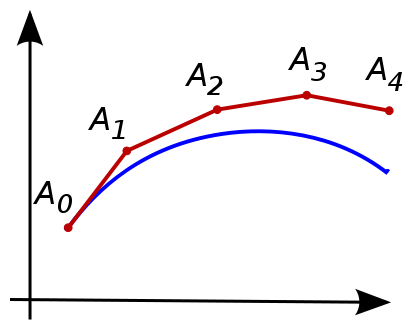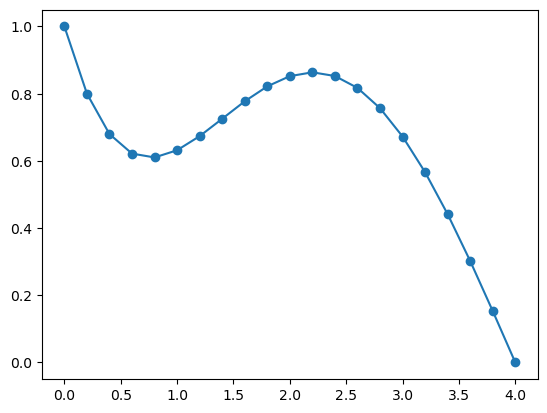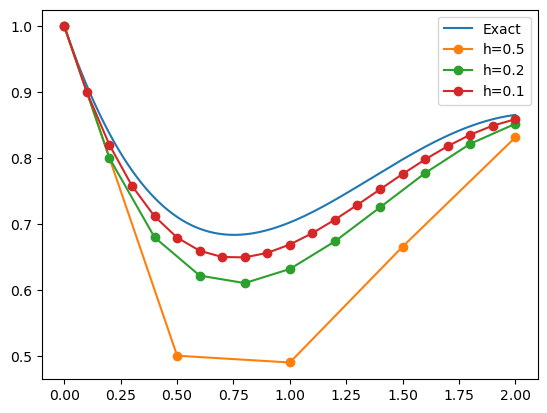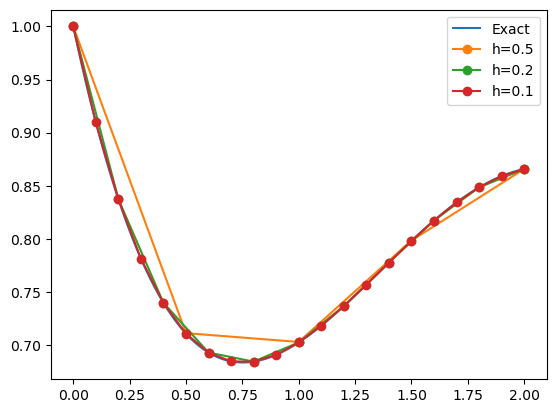# 14.1. Initial Value Problems¶

An initial value problem (IVP) can be written as:

\begin{split} \begin{align*} y'(t) &= f(t,y(t)), \quad t\ge t_0 \\ y(t_0) &= y_0 \end{align*} \end{split}

for some right-hand side function $$f(t,y)$$ and initial condition $$y_0$$ for $$t=t_0$$. In these problems, the independent variable $$t$$ often represents time.

Sometimes basic calculus can be used to solve these equations. For example, some linear and separable equations have closed-form solutions. But in general we have to resort to numerical methods to find approximations to the solution $$y(t)$$.

using PyPlot, PyCall


## 14.1.1. Euler’s method¶

The most basic approach for numerically solving an IVP is Euler’s method, illustrated below.(from Wikipedia, https://en.wikipedia.org/wiki/Euler_method).

We first choose a step size, or time step, $$h$$, and define the discrete times $$t_n = t_0 + nh$$, $$n = 0,1,\ldots$$. Euler’s method advances the solution $$y_n$$ at time $$t_n$$ to time $$t_{n+1}$$ by a linear approximation using the derivative at time $$t_n$$:

$y_{n+1} = y_n + hf(t_n,y_n)$

With some assumptions on the IVP and the step size, these values can be shown to be good approximation to the true solution, that is, $$y_n \approx y(t_n$$).

While described for scalar-valued functions $$y(t)$$, the method works equally well for systems of equations, that is, when $$y(t)$$ and $$f(t,y(t))$$ are vector-valued.

## 14.1.2. Euler’s method, implementation¶

We implement a general version of Euler’s method which takes the right-hand side function $$f(t,y(t))$$ as an argument. In addition, it takes the initial condition $$y_0$$, the step size $$h$$, the number of steps $$N$$, and the starting time $$t_0$$ which has a default value of $$0$$. Note that the implementations supports solutions $$y$$ that are vector-valued, and the output y is a 2D array of the approximate solutions at each time step.

function euler(f, y0, h, N, t0=0.0)
t = t0 .+ h*(0:N)
y = zeros(N+1, length(y0))

y[1,:] .= y0
for n = 1:N
y[n+1,:] = y[n,:] + h * f(t[n], y[n,:])
end

return t,y
end

euler (generic function with 2 methods)


We demonstrate the method on a model problem with $$f(t,y) = -y + \sin t$$ and $$y(0) = 1$$. We solve using Euler’s method with time step $$h=0.2$$ and $$N=20$$ steps.

f(t,y) = -y .+ sin(t)
t,y = euler(f, 1, 0.2, 20)
plot(t, y, "-o");We can compare the results to the exact solution:

$y_\mathrm{exact} = e^{-t} + (\sin t - \cos t + e^{-t}) / 2$

In the code below, we solve three times using various time steps $$h$$. In the plot, we see that the results are generally better for smaller $$h$$ (as expected), and the accuracy seems to roughly scale linearly with $$h$$. This can be shown to be true in general: the method is convergent (the approximations approach the exact solution as $$h \rightarrow 0$$), and the method is first-order accurate (the error scales with the first power of $$h$$).

yexact(t) = exp(-t) + (sin(t) - cos(t) + exp(-t)) / 2
tt = 0:0.01:2
plot(tt, yexact.(tt))
for h = [0.5, 0.2, 0.1]
t,y = euler(f, 1, h, round(Int, 2/h))
plot(t, y, "-o")
end
legend(("Exact", "h=0.5", "h=0.2", "h=0.1"));## 14.1.3. The Runge-Kutta method¶

While Euler’s method does give accurate results for small enough time steps $$h$$, its first-order convergence is often considered too slow and much better methods have been developed. One of the most popular methods is the following 4th order accurate Runge-Kutta method (RK4):

\begin{split} \begin{align*} k_1 &= h f(t_n, y_n) \\ k_2 &= h f(t_n + h/2, y_n + k_1/2) \\ k_3 &= h f(t_n + h/2, y_n + k_2/2) \\ k_4 &= h f(t_n + h, y_n + k_3) \\ y_{n+1} &= y_n + (k_1 + 2k_2 + 2k_3 + k_4) / 6 \end{align*} \end{split}

We implement it in a very similar way to before. Note that each step now requires computing the four so-called stages or stage derivatives $$k_1,k_2,k_3,k_4$$.

function rk4(f, y0, h, N, t0=0)
t = t0 .+ h*(0:N)
y = zeros(N+1, length(y0))

y[1,:] .= y0
for n = 1:N
k1 = h * f(t[n], y[n,:])
k2 = h * f(t[n] + h/2, y[n,:] + k1/2)
k3 = h * f(t[n] + h/2, y[n,:] + k2/2)
k4 = h * f(t[n] + h, y[n,:] + k3)
y[n+1,:] = y[n,:] + (k1 + 2k2 + 2k3 + k4) / 6
end

return t,y
end

rk4 (generic function with 2 methods)


Run the same test problem as before using RK4:

yexact(t) = exp(-t) + (sin(t) - cos(t) + exp(-t)) / 2
tt = 0:0.01:2
plot(tt, yexact.(tt))
for h = [0.5, 0.2, 0.1]
t,y = rk4(f, 1, h, round(Int, 2/h))
plot(t, y, "-o")
end
legend(("Exact", "h=0.5", "h=0.2", "h=0.1"));We can see that the results are fundamentally more accurate. In fact, even with largest time step $$h=0.5$$, the point values are essentially right on top of the exact solution. We can compute the error for the smallest time step $$h=0.1$$, and note that it has 6 accurate digits:

errors = @. y - yexact(t)
max_error = maximum(abs.(errors))

5.015261516083669e-7